Next: Example Up: Implementation without gradient Previous: The CoeffLinear procedure   Contents

Using an expansion

In some case it may be interesting to consider an expansion of the function around a given point. For example consider the term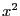with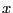in the range [1,2]: in the simplex method the range [1,4], for this term will be added to the non linear part of the equation. But if we substitute the unknownby a new unknown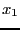such that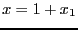(hence the range forwill be [0,1]) we will get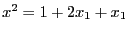we will get an additional linear term (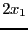) while the non linear part will be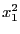with the range [0,1]. For each variable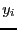in the range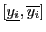we may define a new variable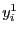such that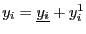wherehas the range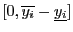. We may then write the non linear and linear procedures for the unknownsbut it necessary to notify the simplex procedure that such an expansion is used. This is done by setting the flag ALIAS_Simplex_Expanded to 1 (this possibility is available only for the simplex method using the gradient).

You may also prohibit the use of the simplex method in the procedure (for example to use it only in your own simplification procedure) by setting the flag ALIAS_DONT_USE_SIMPLEX to 1.Next: Example Up: Implementation without gradient Previous: The CoeffLinear procedure   Contents
Jean-Pierre Merlet 2012-12-20